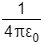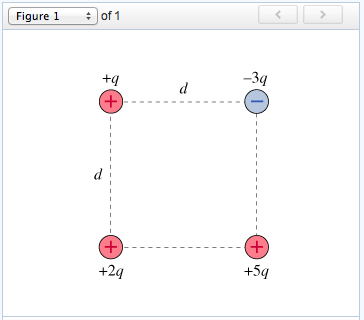# Problem: Four point charges form a square with sides of length d, as shown in the figure. In the questions that follow, use the constant k in place of .What will be the potential energy Utot of the system of charges when charge +2q is at a very large distance from the other charges? Express your answer in terms of q, d, and appropriate constants.

###### FREE Expert Solution

Electric potential energy:

$\overline{){\mathbf{U}}{\mathbf{=}}\frac{\mathbf{k}{\mathbf{q}}_{\mathbf{1}}{\mathbf{q}}_{\mathbf{2}}}{\mathbf{r}}}$

When the charge 2q is far away, the system potential is contributed by 3 charges.

rq,-3q = d

r5q,-3q = d

rq,5q = (d2 + d2)(1/2) = 2(1/2)d

99% (392 ratings)###### Problem Details

Four point charges form a square with sides of length d, as shown in the figure. In the questions that follow, use the constant k in place of.What will be the potential energy Utot of the system of charges when charge +2q is at a very large distance from the other charges?